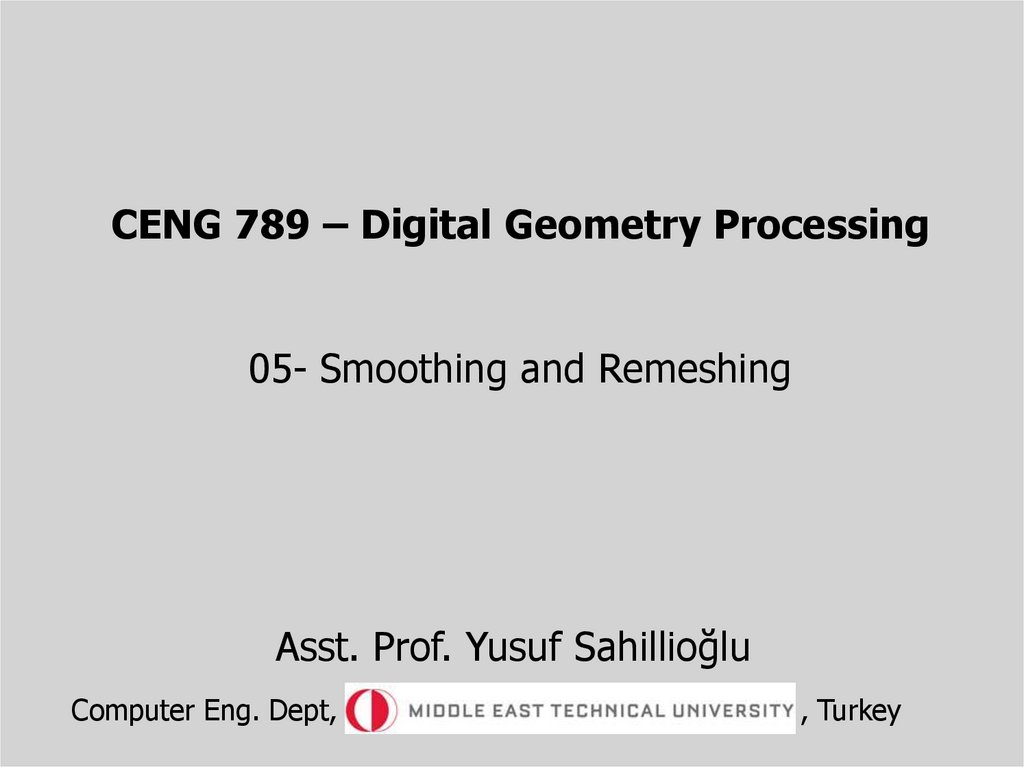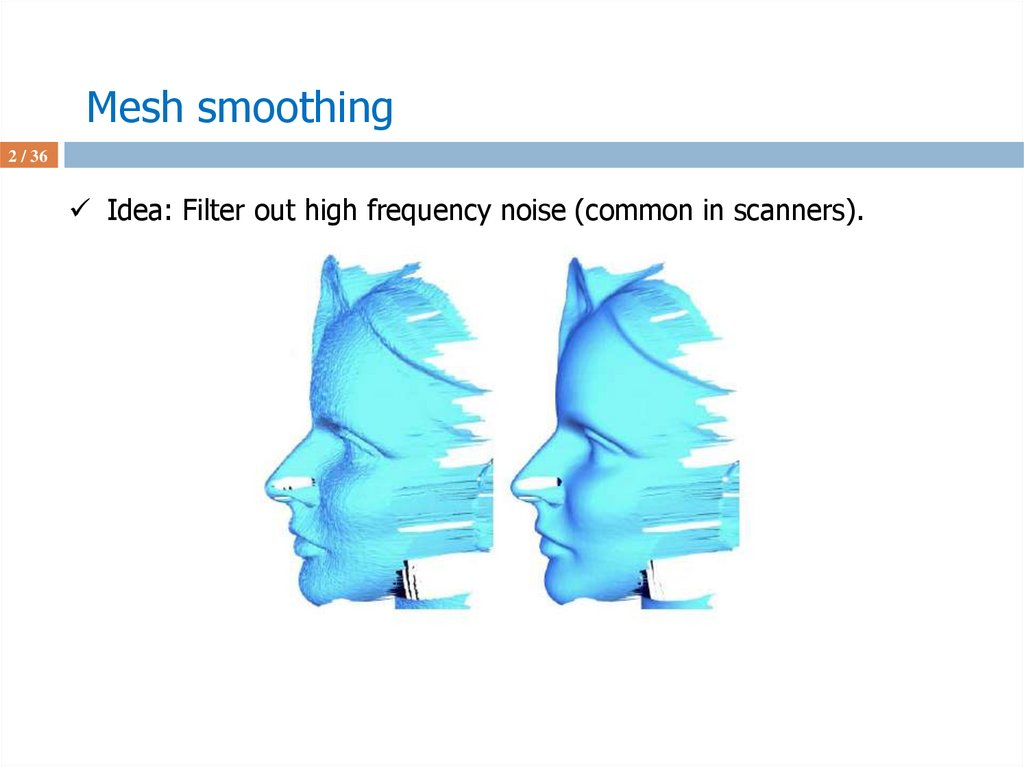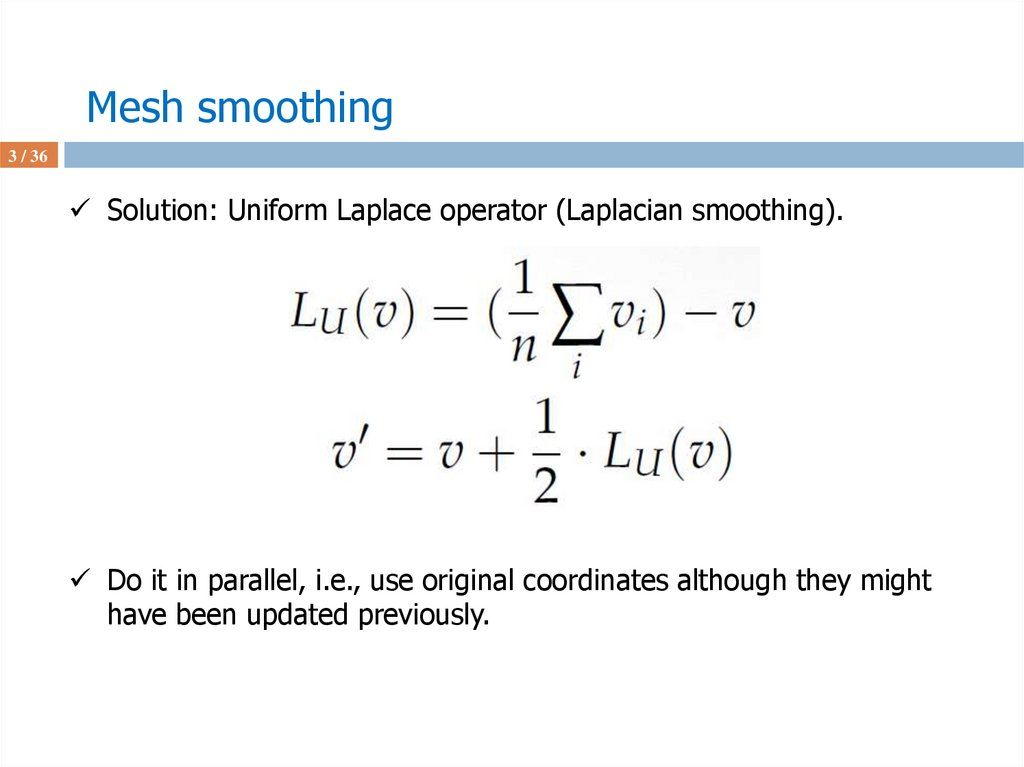# CENG 789 – Digital Geometry Processing

## 1.

CENG 789 – Digital Geometry Processing
05- Smoothing and Remeshing
Asst. Prof. Yusuf Sahillioğlu
Computer Eng. Dept,
, Turkey

## 2. Mesh smoothing

2 / 36
Idea: Filter out high frequency noise (common in scanners).

## 3. Mesh smoothing

3 / 36
Solution: Uniform Laplace operator (Laplacian smoothing).
Do it in parallel, i.e., use original coordinates although they might
have been updated previously.

## 4. Mesh smoothing

4 / 36
Illustration in 1D:

## 5. Mesh smoothing

5 / 36
Illustration in 1D:

## 6. Mesh smoothing

6 / 36
Observation: close curve converges to a single point?

## 7. Mesh smoothing

7 / 36
Illustration in 2D: Same as for curves (1D).

## 8. Mesh smoothing

8 / 36
Observation: close mesh, e.g., sphere, converges to a single point.

## 9. Mesh smoothing

9 / 36
Observation: shrinkage problem.
Repeated iterations of Laplacian smoothing shrinks the mesh.

## 10. Mesh smoothing

10 / 36
Solution: shrinkage problem is remedied with an inflation term.
This is introduced by the Mesh Fairing paper by Taubin in 1995.

## 11. Remeshing

11 / 36
Given a 3D mesh, compute another mesh, whose elements satisfy
some quality requirements, while approximating the input acceptably.
In short, mesh quality improvement.
In contrast to mesh repair (next class), the input of remeshing
algorithms is usually assumed to be a manifold triangle mesh.
Mesh quality: sampling density, regularity, and shape of mesh
elements.

12 / 36

## 13. Remeshing

13 / 36
cleaner
edge directions not messy

## 14. Remeshing

14 / 36
Favoring triangle meshes.
Four points or more may not be on the same plane, but three points always
are (ignoring collinearity). This has the interesting property that scalar values
vary linearly over the surface of the triangle.
This, in turn, means most if not all of what's needed to shade, texture map,
and depth filter a triangle can be calculated using linear interpolation which
can be done extremely fast in specialized hardware.
Triangles are the simplest* primitive, so algorithms dealing with triangles can
be heavily optimized, e.g., fast point-in-triangle test.
* every object can be split into triangles but a triangle cannot be split into
anything else than triangles.

15 / 36

## 16. Remeshing

16 / 36
Mesh elements: shape: isotropic vs. anisotropic.
The shape of isotropic elements is locally uniform in all directions.
Ideally, a triangle/quadrangle is isotropic if it is close to
equilateral/square.

## 17. Remeshing

17 / 36
Mesh elements: shape: isotropic vs. anisotropic.
The shape of isotropic elements is locally uniform in all directions.
Ideally, a triangle/quadrangle is isotropic if it is close to
equilateral/square.
Isotropic elements: roundness ~ 1. (favored in numerical apps, FEM).
Roundness: ratio of circumcircle radius to the length of the shortest
edge.

## 18. Remeshing

18 / 36
Mesh elements: sampling: uniform vs. adaptive.
Smaller elements are assigned to areas w/ high curvature.

## 19. Remeshing

19 / 36
Mesh elements: sampling: uniform vs. adaptive.
Smaller elements are assigned to areas w/ high curvature.

## 20. Remeshing

20 / 36
Mesh elements: regularity: irregular vs. regular.
Valence close to 6; ~equal edge lengths.

## 21. Remeshing

21 / 36
Remeshing approaches: parametrization-based vs. surface-based.
Param-based: map to 2D domain, do the remeshing (2d problem), lift
up.

## 22. Remeshing

22 / 36
Remeshing approaches: parametrization-based vs. surface-based.
Param-based: map to 2D domain, do the remeshing (2d problem), lift
up.
Delaunay triangulation: maximize the min angle = no point in
circumcircle of a triangle.

## 23. Remeshing

23 / 36
Remeshing approaches: parametrization-based vs. surface-based.
surface-based: work directly on the mesh embedded in 3D.

## 24. Remeshing

24 / 36
Remeshing approaches: parametrization-based vs. surface-based.
surface-based: work directly on the mesh embedded in 3D.
Beware of illegal edge collapses:
i) Normal flip after collapse!
ii) intersection of 1-ring
neighborhood of i and j
contains 3+ vertices!

## 25. Remeshing

25 / 36
Remeshing approaches: parametrization-based vs. surface-based.
surface-based: work directly on the mesh embedded in 3D.
Beware of illegal edge collapses:
i) A heuristic while removing short edges:
Collapse into the vert w/ higher valence.
Works ‘cos high-valence verts stay fixed and
every collapse reduces # adjacent short edges.

## 26. Remeshing

26 / 36
Remeshing approaches: parametrization-based vs. surface-based.
surface-based: work directly on the mesh embedded in 3D.
Beware of illegal edge splits:
i) Infinite-loop problem if you split shorter edges first (top row)!

## 27. Remeshing

27 / 36
Remeshing approaches: parametrization-based vs. surface-based.
surface-based: work directly on the mesh embedded in 3D.
Beware of illegal edge flips:
i) edge is adjacent to 2 tris whose union is not a convex quadrilateral!
convex if no projection (of the 4th vert) is inside the tri (defined by the
other 3 verts) //4th vert is projected to the plane defined by the other 3.

## 28. Remeshing

28 / 36
Remeshing approaches: parametrization-based vs. surface-based.
surface-based: work directly on the mesh embedded in 3D.
A sequence of edge collapses, aka mesh decimation:

## 29. Remeshing

29 / 36
Remeshing approaches: parametrization-based vs. surface-based.
surface-based: work directly on the mesh embedded in 3D.
A sequence of edge collapses, aka mesh decimation:
Isosurface extraction by Marching Cubes over-tessellates (left).

## 30. Remeshing

30 / 36
Metric for edge collapses (other than edge length metric).
Curvature factor is introduced as coplanar surfaces can be represented
using fewer polygons than areas w/ a high curvature.
Good collapses:

## 31. Remeshing

31 / 36
Metric for edge collapses (other than edge length metric).
Curvature factor is introduced as coplanar surfaces can be represented
using fewer polygons than areas w/ a high curvature.
Collapse cost of edge (u,v): Tu is the set of triangles that contain the
vertex u and Tuv is the set of triangles that share the edge (u,v).
Cost is length (||u-v||) multiplied by a curvature factor (< 1).
Curvature factor computed by comparing the dot products of all
involved face normals to find the largest angle b/w 2 faces.

## 32. Remeshing

32 / 36
Metric for edge collapses (other than edge length metric).
Error quadric: based on the observation that in the original model
each vertex is the solution of the intersection of a set of planes.
Such a set of planes is associatd w/ each vertex as supporting planes.
The error at the vertex w.r.t. this set is the sum of squared distances
to its supporting planes.
Hence this error helps preserving the original details in the decimated
model.
Edge-length metric:

## 33. Remeshing

33 / 36
Metric for edge collapses (other than edge length metric).
Error quadric in 1D (supporting planes supporting lines).

## 34. Remeshing

34 / 36
Metric for edge collapses (other than edge length metric).
Error quadric Kp can be used to find the squared distance of any point in space to its supporting planes.

## 35. Remeshing

35 / 36
Metric for edge collapses (other than edge length metric).

## 36. Remeshing

36 / 36
Metric for edge collapses (other than edge length metric).
Algorithm:
Collapse cost of edge (u,v): compute the optimal contraction target vertex for
this edge (for simplicity u collapses to v). The error at this proposed new
vertex (v) becomes the cost of collapsing this edge.
Place all edges in a min-heap keyed on collapse costs.
Iteratively remove the edge w/ min cost, collapse it, update the costs of all
involved edges.
Detail: original algorithm uses ‘vertex pairs’ = edges + 2-close-vertices.
Detail: original algorithm collapses u to a point p* that minimizes error Kp*.
Surface Simplification Using Quadric Error Metrics.

## 37. Remeshing

37 / 36
Term project ideas.
Normal orientation correction: naïve algo (neighbor triangles have similar